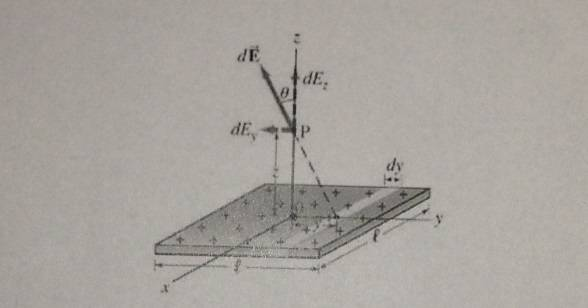# Uniform plane of charge

## Homework Statement[/B]
Charge is distributed uniformly over a large square plane of side l, as shown in the figure. The charge per unit area (C/m^2) is $\sigma$. Determine the electric field at a point P a distance z above the center of the plane, in the limit $l \to \infty$.
[Hint: Divide the plane into long narrow strips of width dy, and use the result of Example 11]

## Homework Equations

[/B]
Result of Example 11: $\frac{2k\lambda }{x}$ (electric field at a distance x due to an infinitely long wire)(that point is symmetric about the x-axis, so there is no y component of the electric field.)

$$k = \frac{1}{4\pi\epsilon_0}$$

## The Attempt at a Solution

Charge densities:
$$\sigma = \frac{dq}{dy*l}$$ (an infinitely small q over an infinitely small surface)
$$\lambda = \frac{dq}{l}$$ (total charge of the strip / total length)

$$dE = \frac{2k\lambda}{\sqrt{y^2+z^2}}$$
(electric field due to a long strip)
$$dE_z = dE sin\theta = \frac{2k\lambda y}{{(y^2+z^2)}^{3/2}}$$
(its z component is what we need)
$$dE_z = dE sin\theta = \frac{2k\sigma y}{{(y^2+z^2)}^{3/2}}dy$$
(dy is necessary, so replace lambda with sigma)

The following is what I get after the integration,

$${-2\sigma k} \frac{1}{\sqrt {y^2+z^2}}$$

The limits are zero and infinity, so I end up with;
$$\frac{2\sigma k}{z}$$

There is an example of uniformly charged disk in my textbook. The formula for electric field for that disk does not depend on the distance. That's why I believe I've done this question wrong. What do you think about my solution? I am not sure if I wrote charge densities correct, so that may be the mistake.

Last edited:

## Answers and Replies

TSny
Homework Helper
Gold Member
When getting the z-component you used a factor of sinθ. Is that correct?

When getting the z-component you used a factor of sinθ. Is that correct?
My bad... It should be cosine, shouldn't it?

TSny
Homework Helper
Gold Member
Yes, that's right.

TSny
Homework Helper
Gold Member
Also, should you end up with a power of 3/2 in the denominator of the integrand?

Also, should you end up with a power of 3/2 in the denominator of the integrand?

It must be raised to the first power I suppose, right?

TSny
Homework Helper
Gold Member
Yes. What should be the limits on the integral in order to cover the entire plate?

Yes. What should be the limits on the integral in order to cover the entire plate?
I know we are doing good right now but I am not good at identifying the limits. Probably wrong, but I would say 0 and l.

TSny
Homework Helper
Gold Member
You are integrating over strips. Where is the strip corresponding to y = 0? As y varies between 0 and ##l##, what strips are included?

You are integrating over strips. Where is the strip corresponding to y = 0? As y varies between 0 and ##l##, what strips are included?

Then I guess -l/2 & l/2 would be the limits, or simply 2 * [0 to l/2]?

TSny
Homework Helper
Gold Member
Yes. Good. ( I should have said as y varies from 0 to ##l/2## above.)

So, as ##l## goes to infinity, you can see what the limits on the integral should be.

•hitemup